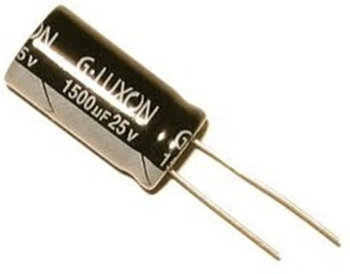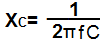﻿ Capacitive Reactance

# Capacitive ReactanceCapacitive reactance is how the impedance (or resistance) of a capacitor changes in regard to the frequency of the signal passing through it.

Capacitors, unlike resistors, are reactive devices. This means that they offer different resistances to signals of differing frequencies. To low-frequency signals, capacitors offer a lot of resistance, so that low-frequency signals are essentially blocked from passing through a capacitor. As the frequencies of the signals passing through a capacitor increase, the capacitor offers less and less resistance, so that higher frequency signals can pass through easier.

The formula for calculating capacitive reactance is:XC is equal to the value of the capacitor reactance or impedance.

You can see, according to this formula, how capacitive reactance changes according to the frequency of the signal input into it. It has an indirect relationship with the frequency of the signal passing through it. The higher the frequency of the signal, the lower the value of the reactance. The lower the frequency of the signal, the higher the value of the reactance.

Related Resources

Capacitor Impedance Calculator

Capacitance Calculator